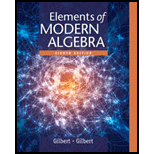# Prove that R contains an idempotent element if and only if R '### Elements Of Modern Algebra

8th Edition
Gilbert + 2 others
Publisher: Cengage Learning,
ISBN: 9781285463230

#### Solutions

Chapter
Section### Elements Of Modern Algebra

8th Edition
Gilbert + 2 others
Publisher: Cengage Learning,
ISBN: 9781285463230
Chapter 6.2, Problem 4E
Textbook Problem
1 views

## Prove that R contains an idempotent element if and only if R '

To determine

To prove: R contains an idempotent element if and only if R' contains an idempotent element.

### Explanation of Solution

Given information:

R and R' are isomorphic arbitrary rings.

Formula used:

i) Idempotent element:

An element x in a ring is called idempotent if x2=x.

ii) Isomorphism:

Let θ be a homomorphism from the ring R to the ring R'. If θ is a one-to-one correspondence (both onto and one-one), then θ is an isomorphism.

Proof:

Let R and R' be isomorphic arbitrary rings.

Therefore, there exists an isomorphism θ:RR' such that

i) θ is a homomorphism from R to R' ;

ii) θ is a one-to-one correspondence (both onto and one-one).

To prove: a) R contains an idempotent element b) R' contains an idempotent element.

a) b): Suppose R contains an idempotent element.

Let a be the idempotent element in R.

a2=a

Now, let θ(a)R' be any element with aR.

θ(a2)=θ(a)

Since θ(a2)=θ(aa) and θ preserve multiplication.

θ(a2)=θ(aa)=θ(a)θ(a)=(θ(a))2

(θ(a))2=θ(a), for aR

### Still sussing out bartleby?

Check out a sample textbook solution.

See a sample solution

#### The Solution to Your Study Problems

Bartleby provides explanations to thousands of textbook problems written by our experts, many with advanced degrees!

Get Started

Find more solutions based on key concepts
In Exercises 1728, use the logarithm identities to obtain the missing quantity.

Finite Mathematics and Applied Calculus (MindTap Course List)

Solve each equation: x26x=0

Elementary Technical Mathematics

a. Let f, g, and h be functions. How would you define the "sum of f, g, and h? b. Give a real-life example invo...

Applied Calculus for the Managerial, Life, and Social Sciences: A Brief Approach

Simplify Simplify the rational expression. 22. y23y182y2+7y+3

Precalculus: Mathematics for Calculus (Standalone Book)

The y-coordinate of the center of mass of the region bounded by , x = 1, x = 5, y = 0 is: 4

Study Guide for Stewart's Single Variable Calculus: Early Transcendentals, 8th

What is the goal of a single-case experimental research design?

Research Methods for the Behavioral Sciences (MindTap Course List)